## Monday, January 25, 2021

### NCERT Class 9 Maths Chapter 8 – Quadrilaterals

NCERT Class 9 Maths Chapter 8 – Quadrilaterals

## Exercise 8.2 Page: 150

1. ABCD is a quadrilateral in which P, Q, R and S are mid-points of the sides AB, BC, CD and DA (see Fig 8.29). AC is a diagonal. Show that:
(i) SR || AC and SR = 1/2 AC
(ii) PQ = SR
(iii) PQRS is a parallelogram.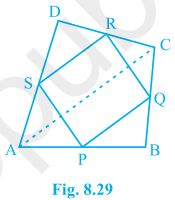Solution:

(i) In ΔDAC,

R is the mid point of DC and S is the mid point of DA.

Thus by mid point theorem, SR || AC and SR = ½ AC

(ii) In ΔBAC,

P is the mid point of AB and Q is the mid point of BC.

Thus by mid point theorem, PQ || AC and PQ = ½ AC

also, SR = ½ AC

, PQ = SR

(iii) SR || AC ———————- from question (i)

and, PQ || AC ———————- from question (ii)

⇒ SR || PQ – from (i) and (ii)

also, PQ = SR

, PQRS is a parallelogram.

2. ABCD is a rhombus and P, Q, R and S are the mid-points of the sides AB, BC, CD and DA respectively. Show that the quadrilateral PQRS is a rectangle.

Solution: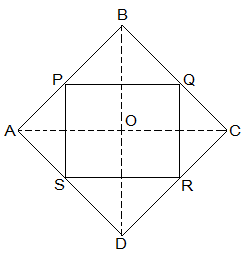Given in the question,

ABCD is a rhombus and P, Q, R and S are the mid-points of the sides AB, BC, CD and DA respectively.

To Prove,

PQRS is a rectangle.

Construction,

Join AC and BD.

Proof:

In ΔDRS and ΔBPQ,

DS = BQ (Halves of the opposite sides of the rhombus)

∠SDR = ∠QBP (Opposite angles of the rhombus)

DR = BP (Halves of the opposite sides of the rhombus)

, ΔDRS ≅ ΔBPQ [SAS congruency]

RS = PQ [CPCT]———————- (i)

In ΔQCR and ΔSAP,

RC = PA (Halves of the opposite sides of the rhombus)

∠RCQ = ∠PAS (Opposite angles of the rhombus)

CQ = AS (Halves of the opposite sides of the rhombus)

, ΔQCR ≅ ΔSAP [SAS congruency]

RQ = SP [CPCT]———————- (ii)

Now,

In ΔCDB,

R and Q are the mid points of CD and BC respectively.

⇒ QR || BD

also,

P and S are the mid points of AD and AB respectively.

⇒ PS || BD

⇒ QR || PS

, PQRS is a parallelogram.

also, ∠PQR = 90°

Now,

In PQRS,

RS = PQ and RQ = SP from (i) and (ii)

∠Q = 90°

, PQRS is a rectangle.

3. ABCD is a rectangle and P, Q, R and S are mid-points of the sides AB, BC, CD and DA respectively. Show that the quadrilateral PQRS is a rhombus.

Solution:Given in the question,

ABCD is a rectangle and P, Q, R and S are mid-points of the sides AB, BC, CD and DA respectively.

Construction,

Join AC and BD.

To Prove,

PQRS is a rhombus.

Proof:

In ΔABC

P and Q are the mid-points of AB and BC respectively

, PQ || AC and PQ = ½ AC (Midpoint theorem) — (i)

SR || AC and SR = ½ AC (Midpoint theorem) — (ii)

So, PQ || SR and PQ = SR

As in quadrilateral PQRS one pair of opposite sides is equal and parallel to each other, so, it is a parallelogram.

, PS || QR and PS = QR (Opposite sides of parallelogram) — (iii)

Now,

In ΔBCD,

Q and R are mid points of side BC and CD respectively.

, QR || BD and QR = ½ BD (Midpoint theorem) — (iv)

AC = BD (Diagonals of a rectangle are equal) — (v)

From equations (i), (ii), (iii), (iv) and (v),

PQ = QR = SR = PS

So, PQRS is a rhombus.

Hence Proved

4. ABCD is a trapezium in which AB || DC, BD is a diagonal and E is the mid-point of AD. A line is drawn through E parallel to AB intersecting BC at F (see Fig. 8.30). Show that F is the mid-point of BC.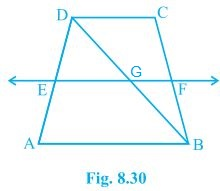Solution:

Given that,

ABCD is a trapezium in which AB || DC, BD is a diagonal and E is the mid-point of AD.

To prove,

F is the mid-point of BC.

Proof,

BD intersected EF at G.

E is the mid point of AD and also EG || AB.

Thus, G is the mid point of BD (Converse of mid point theorem)

Now,

In ΔBDC,

G is the mid point of BD and also GF || AB || DC.

Thus, F is the mid point of BC (Converse of mid point theorem)

5. In a parallelogram ABCD, E and F are the mid-points of sides AB and CD respectively (see Fig. 8.31). Show that the line segments AF and EC trisect the diagonal BD.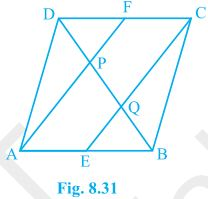Solution:

Given that,

ABCD is a parallelogram. E and F are the mid-points of sides AB and CD respectively.

To show,

AF and EC trisect the diagonal BD.

Proof,

ABCD is a parallelogram

, AB || CD

also, AE || FC

Now,

AB = CD (Opposite sides of parallelogram ABCD)

⇒½ AB = ½ CD

⇒ AE = FC (E and F are midpoints of side AB and CD)

AECF is a parallelogram (AE and CF are parallel and equal to each other)

AF || EC (Opposite sides of a parallelogram)

Now,

In ΔDQC,

F is mid point of side DC and FP || CQ (as AF || EC).

P is the mid-point of DQ (Converse of mid-point theorem)

⇒ DP = PQ — (i)

Similarly,

In ΔAPB,

E is midpoint of side AB and EQ || AP (as AF || EC).

Q is the mid-point of PB (Converse of mid-point theorem)

⇒ PQ = QB — (ii)

From equations (i) and (i),

DP = PQ = BQ

Hence, the line segments AF and EC trisect the diagonal BD.

Hence Proved.

6Show that the line segments joining the mid-points of the opposite sides of a quadrilateral bisect each other.

Solution: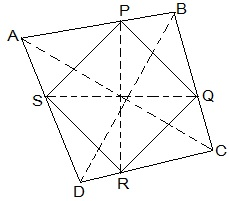Let ABCD be a quadrilateral and P, Q, R and S are the mid points of AB, BC, CD and DA respectively.

Now,

In ΔACD,

R and S are the mid points of CD and DA respectively.

, SR || AC.

Similarly we can show that,

PQ || AC,

PS || BD and

QR || BD

, PQRS is parallelogram.

PR and QS are the diagonals of the parallelogram PQRS. So, they will bisect each other.

7. ABC is a triangle right angled at C. A line through the mid-point M of hypotenuse AB and parallel to BC intersects AC at D. Show that
(i) D is the mid-point of AC
(ii) MD ⊥ AC
(iii) CM = MA = ½ AB

Solution: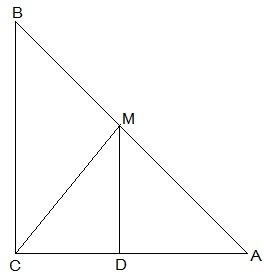(i) In ΔACB,

M is the midpoint of AB and MD || BC

, D is the midpoint of AC (Converse of mid point theorem)

(ii) ∠ACB = ∠ADM (Corresponding angles)

also, ∠ACB = 90°

, ∠ADM = 90° and MD ⊥ AC

(iii) In ΔAMD and ΔCMD,

AD = CD (D is the midpoint of side AC)

DM = DM (common)

, ΔAMD ≅ ΔCMD [SAS congruency]

AM = CM [CPCT]

also, AM = ½ AB (M is midpoint of AB)

Hence, CM = MA = ½ AB

### NCERT Class 9 Maths Chapter 8 – Quadrilaterals

NCERT Class 9 Maths Chapter 8 – Quadrilaterals

# NCERT Class 9 Maths Chapter 8 – Quadrilaterals

## Exercise 8.1 Page: 146

#### 1. The angles of quadrilateral are in the ratio 3 : 5 : 9 : 13. Find all the angles of the quadrilateral.

Solution:

Let the common ratio between the angles be = x.

We know that the sum of the interior angles of the quadrilateral = 360°

Now,

3x+5x+9x+13x = 360°

⇒ 30x = 360°

⇒ x = 12°

, Angles of the quadrilateral are:

3x = 3×12° = 36°

5x = 5×12° = 60°

9x = 9×12° = 108°

13x = 13×12° = 156°

2. If the diagonals of a parallelogram are equal, then show that it is a rectangle.

Solution: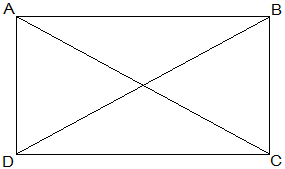Given that,

AC = BD

To show that, ABCD is a rectangle if the diagonals of a parallelogram are equal

To show ABCD is a rectangle we have to prove that one of its interior angles is right angled.

Proof,

BC = BA (Common)

AC = AD (Opposite sides of a parallelogram are equal)

AC = BD (Given)

Therefore, ΔABC ≅ ΔBAD [SSS congruency]

∠A = ∠B [Corresponding parts of Congruent Triangles]

also,

∠A+∠B = 180° (Sum of the angles on the same side of the transversal)

⇒ 2∠A = 180°

⇒ ∠A = 90° = ∠B

, ABCD is a rectangle.

Hence Proved.

3. Show that if the diagonals of a quadrilateral bisect each other at right angles, then it is a rhombus.

Solution: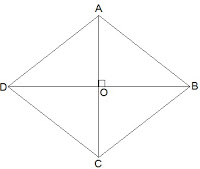Let ABCD be a quadrilateral whose diagonals bisect each other at right angles.

Given that,

OA = OC

OB = OD

and ∠AOB = ∠BOC = ∠OCD = ∠ODA = 90°

To show that,

if the diagonals of a quadrilateral bisect each other at right angles, then it is a rhombus.

i.e., we have to prove that ABCD is parallelogram and AB = BC = CD = AD

Proof,

In ΔAOB and ΔCOB,

OA = OC (Given)

∠AOB = ∠COB (Opposite sides of a parallelogram are equal)

OB = OB (Common)

Therefore, ΔAOB ≅ ΔCOB [SAS congruency]

Thus, AB = BC [CPCT]

Similarly we can prove,

BC = CD

, AB = BC = CD = AD

Opposites sides of a quadrilateral are equal hence ABCD is a parallelogram.

, ABCD is rhombus as it is a parallelogram whose diagonals intersect at right angle.

Hence Proved.

4. Show that the diagonals of a square are equal and bisect each other at right angles.

Solution: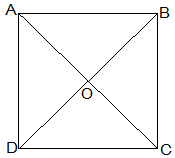Let ABCD be a square and its diagonals AC and BD intersect each other at O.

To show that,

AC = BD

AO = OC

and ∠AOB = 90°

Proof,

AB = BA (Common)

Thus,

AC = BD [CPCT]

diagonals are equal.

Now,

In ΔAOB and ΔCOD,

∠BAO = ∠DCO (Alternate interior angles)

∠AOB = ∠COD (Vertically opposite)

AB = CD (Given)

, ΔAOB ≅ ΔCOD [AAS congruency]

Thus,

AO = CO [CPCT].

, Diagonal bisect each other.

Now,

In ΔAOB and ΔCOB,

OB = OB (Given)

AO = CO (diagonals are bisected)

AB = CB (Sides of the square)

, ΔAOB ≅ ΔCOB [SSS congruency]

also, ∠AOB = ∠COB

∠AOB+∠COB = 180° (Linear pair)

Thus, ∠AOB = ∠COB = 90°

, Diagonals bisect each other at right angles

5. Show that if the diagonals of a quadrilateral are equal and bisect each other at right angles, then it is a square.

Solution: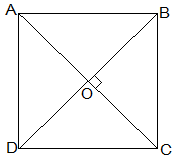Given that,

Let ABCD be a quadrilateral and its diagonals AC and BD bisect each other at right angle at O.

To prove that,

The Quadrilateral ABCD is a square.

Proof,

In ΔAOB and ΔCOD,

AO = CO (Diagonals bisect each other)

∠AOB = ∠COD (Vertically opposite)

OB = OD (Diagonals bisect each other)

, ΔAOB ≅ ΔCOD [SAS congruency]

Thus,

AB = CD [CPCT] — (i)

also,

∠OAB = ∠OCD (Alternate interior angles)

⇒ AB || CD

Now,

In ΔAOD and ΔCOD,

AO = CO (Diagonals bisect each other)

∠AOD = ∠COD (Vertically opposite)

OD = OD (Common)

, ΔAOD ≅ ΔCOD [SAS congruency]

Thus,

AD = CD [CPCT] — (ii)

also,

⇒ AD = BC = CD = AB — (ii)

and ∠ADC+∠BCD = 180° (co-interior angles)

One of the interior angles is right angle.

Thus, from (i), (ii) and (iii) given quadrilateral ABCD is a square.

Hence Proved.

6. Diagonal AC of a parallelogram ABCD bisects ∠A (see Fig. 8.19). Show that

(i) it bisects ∠C also,

(ii) ABCD is a rhombus.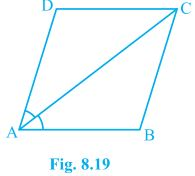Solution:

AD = CB (Opposite sides of a parallelogram)

DC = BA (Opposite sides of a parallelogram)

AC = CA (Common Side)

, ΔADC ≅ ΔCBA [SSS congruency]

Thus,

∠ACD = ∠CAB by CPCT

⇒ ∠ACD = ∠BCA

Thus,

AC bisects ∠C also.

(ii) ∠ACD = ∠CAD (Proved above)

⇒ AD = CD (Opposite sides of equal angles of a triangle are equal)

Also, AB = BC = CD = DA (Opposite sides of a parallelogram)

Thus,

ABCD is a rhombus.

7. ABCD is a rhombus. Show that diagonal AC bisects ∠A as well as ∠C and diagonal BD bisects ∠B as well as ∠D.

Solution:Given that,

ABCD is a rhombus.

AC and BD are its diagonals.

Proof,

AD = CD (Sides of a rhombus)

∠DAC = ∠DCA (Angles opposite of equal sides of a triangle are equal.)

also, AB || CD

⇒∠DAC = ∠BCA (Alternate interior angles)

⇒∠DCA = ∠BCA

, AC bisects ∠C.

Similarly,

We can prove that diagonal AC bisects ∠A.

Following the same method,

We can prove that the diagonal BD bisects ∠B and ∠D.

8. ABCD is a rectangle in which diagonal AC bisects ∠A as well as ∠C. Show that:

(i) ABCD is a square

(ii) Diagonal BD bisects ∠B as well as ∠D.

Solution: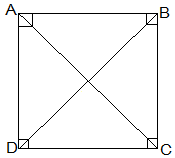(i) ∠DAC = ∠DCA (AC bisects ∠A as well as ∠C)

⇒ AD = CD (Sides opposite to equal angles of a triangle are equal)

also, CD = AB (Opposite sides of a rectangle)

,AB = BC = CD = AD

Thus, ABCD is a square.

(ii) In ΔBCD,

BC = CD

⇒ ∠CDB = ∠CBD (Angles opposite to equal sides are equal)

also, ∠CDB = ∠ABD (Alternate interior angles)

⇒ ∠CBD = ∠ABD

Thus, BD bisects ∠B

Now,

Thus, BD bisects ∠D

9. In parallelogram ABCD, two points P and Q are taken on diagonal BD such that DP = BQ (see Fig. 8.20). Show that:

(i) ΔAPD ≅ ΔCQB

(ii) AP = CQ

(iii) ΔAQB ≅ ΔCPD

(iv) AQ = CP

(v) APCQ is a parallelogram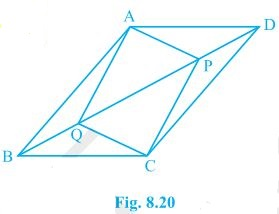Solution:

(i) In ΔAPD and ΔCQB,

DP = BQ (Given)

∠ADP = ∠CBQ (Alternate interior angles)

AD = BC (Opposite sides of a parallelogram)

Thus, ΔAPD ≅ ΔCQB [SAS congruency]

(ii) AP = CQ by CPCT as ΔAPD ≅ ΔCQB.

(iii) In ΔAQB and ΔCPD,

BQ = DP (Given)

∠ABQ = ∠CDP (Alternate interior angles)

AB = CD (Opposite sides of a parallelogram)

Thus, ΔAQB ≅ ΔCPD [SAS congruency]

(iv) As ΔAQB ≅ ΔCPD

AQ = CP [CPCT]

(v) From the questions (ii) and (iv), it is clear that APCQ has equal opposite sides and also has equal and opposite angles. , APCQ is a parallelogram.

10. ABCD is a parallelogram and AP and CQ are perpendiculars from vertices A and C on diagonal BD (see Fig. 8.21). Show that

(i) ΔAPB ≅ ΔCQD

(ii) AP = CQ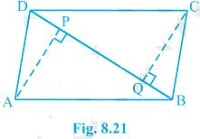Solution:

(i) In ΔAPB and ΔCQD,

∠ABP = ∠CDQ (Alternate interior angles)

∠APB = ∠CQD (= 90o as AP and CQ are perpendiculars)

AB = CD (ABCD is a parallelogram)

, ΔAPB ≅ ΔCQD [AAS congruency]

(ii) As ΔAPB ≅ ΔCQD.

, AP = CQ [CPCT]

11. In ΔABC and ΔDEF, AB = DE, AB || DE, BC = EF and BC || EF. Vertices A, B and C are joined to vertices D, E and F respectively (see Fig. 8.22).

Show that

(i) quadrilateral ABED is a parallelogram

(ii) quadrilateral BEFC is a parallelogram

(iv) quadrilateral ACFD is a parallelogram

(v) AC = DF

(vi) ΔABC ≅ ΔDEF.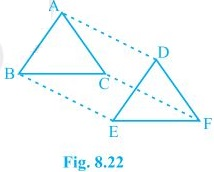Solution:

(i) AB = DE and AB || DE (Given)

Two opposite sides of a quadrilateral are equal and parallel to each other.

Thus, quadrilateral ABED is a parallelogram

(ii) Again BC = EF and BC || EF.

Thus, quadrilateral BEFC is a parallelogram.

(iii) Since ABED and BEFC are parallelograms.

⇒ AD = BE and BE = CF (Opposite sides of a parallelogram are equal)

Also, AD || BE and BE || CF (Opposite sides of a parallelogram are parallel)

(iv) AD and CF are opposite sides of quadrilateral ACFD which are equal and parallel to each other. Thus, it is a parallelogram.

(v) Since ACFD is a parallelogram

AC || DF and AC = DF

(vi) In ΔABC and ΔDEF,

AB = DE (Given)

BC = EF (Given)

AC = DF (Opposite sides of a parallelogram)

, ΔABC ≅ ΔDEF [SSS congruency]

12. ABCD is a trapezium in which AB || CD and AD = BC (see Fig. 8.23). Show that

(i) ∠A = ∠B

(ii) ∠C = ∠D

(iv) diagonal AC = diagonal BD

[Hint : Extend AB and draw a line through C parallel to DA intersecting AB produced at E.]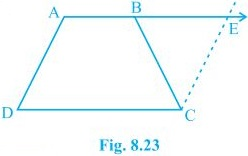Solution:

To Construct: Draw a line through C parallel to DA intersecting AB produced at E.

(i) CE = AD (Opposite sides of a parallelogram)

, BC = CE

⇒∠CBE = ∠CEB

also,

∠A+∠CBE = 180° (Angles on the same side of transversal and ∠CBE = ∠CEB)

∠B +∠CBE = 180° ( As Linear pair)

⇒∠A = ∠B

(ii) ∠A+∠D = ∠B+∠C = 180° (Angles on the same side of transversal)

⇒∠A+∠D = ∠A+∠C (∠A = ∠B)

⇒∠D = ∠C

AB = AB (Common)

∠DBA = ∠CBA

, ΔABC ≅ ΔBAD [SAS congruency]

(iv) Diagonal AC = diagonal BD by CPCT as ΔABC ≅ ΔBA.

____________________________________________________________________________________

## Featured Post